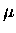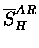Next: Electron scintillator measurements Up: Analysis I - Absolute Previous: Muon stopping fraction determination

# Amplitude ratio method

The first method takes advantage of the fact that the lifetime of the muon is different in hydrogen and gold, being about 2.2s and 70 ns, respectively. The electron time spectrum is measured with a pair of electron scintillation counters surrounding the target chamber (En1, En2, and Ege), and with NE213 neutron detectors (N1 and N2) with their fast dynode output (Tel1 and Tel2) in coincidence with the scintillator pair hit in front of them.

The time of the first hit among En1, En2, or Ege (first E), as well as the first hit of Tel1 and Tel2 (first Tel), are also histogrammed, which if we accept only one muon at a time, and if the effect of background is small, should equal to the sum of En1, En2 and Ege, or Tel1 and Tel2 respectively.

The recorded time spectra were fitted to exponential functions with a constant background. The general form of the fitting function, taking into account capture and other muon losses, as well as the finite size of the time bin, is derived in Eq. 6.4 in Appendix C:

 (84)

where for kth element ( ), is the electron detection efficiency, Qk the Huff factor, N0k the initial muon population, and the muon disappearance rate in element k, with being that in free space. The above is a function of a discrete time variable ti with interval .

Let us consider the specific case of M=2 (two exponentials), where we assume all observed muon decay takes place in one of the two materials. Also we take k=1 for a heavy element such as gold present in the system, and k=2 for hydrogen. We define the fraction of muon stopping in hydrogen SH, hence N01=N0(1-SH) and N02=N0SH. In actual fits, the time histogram is normalized to GMU (number of good muons''), and thus the fit function is defined to give, together with the decay rates , the normalized amplitudes GMU. Hence, the stopping fraction can be determined from the ratio

the superscript AR'' indicating the stopping fraction being derived from the amplitude ratio. We define a reduced stopping fractionas,

 (85)

which would equal S HAR, if we assume (a) Q=1 for hydrogen, and further (b) . The assumption (a) is valid since the binding energy of muonic hydrogen ( keV) is much smaller than the average electron energy ( MeV), but (b) needs careful consideration, which I shall revisit later.Next: Electron scintillator measurements Up: Analysis I - Absolute Previous: Muon stopping fraction determination